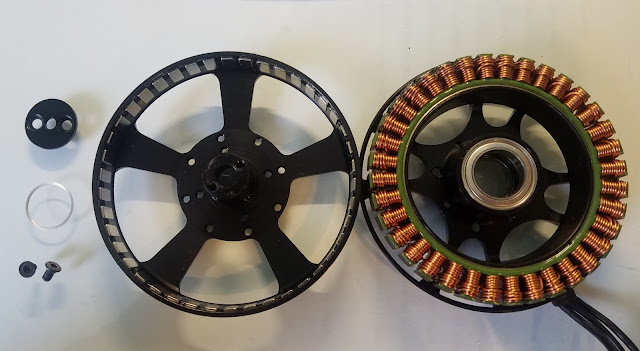## Apr 18, 2017

### [2.70] Seek and Geek #9: U8 Outrunner BLDC Motor

This post is about bearing placement, shaft alignment, and hi-speed cantilever moment stiffness in outrunner brushless motors!A disassembled U8 brushless motor

The point of a motor is to transmit torque, so motor bearings play an integral role in taking on load and minimizing friction losses. This particular motor supports a max thrust of 2.6kg using two bearings with load ratings of 2070N.

Guesstimating at moment resisted by the bearings...
Max stall torque from the motor is 0.912 Nm, and the rotor shaft is pressed on with probably a 5µm tolerance. Assuming no axial loading on the bearings, each bearing experiences half this torque as a radial force = approx. 50N.

We call the distance between bearings $a = 18mm$. F_bearing is the load on each bearing, which includes both applied loads and misalignment loads. By treating this shaft like a cantilever beam, we can calculate forces on the bearings due to misalignment:

$F = kx$,
and
$F_{bearing}\cdot a = K_{moment}\cdot\alpha = \frac{2EI}{L}\cdot \frac{\delta_{tol}}{L}$.
$F_{bearing} = \frac{2EI\delta_{tol}}{aL^2} + F_{applied}$

For this motor, L = 25mm, E = 69 GPa (material assumed to be 6061 AL), and I = 4019mm^4
F_{misalignment} = 247N worst case.

So each bearing experiences 300N at max. torque of the motor, or nominally 15% of load rating.